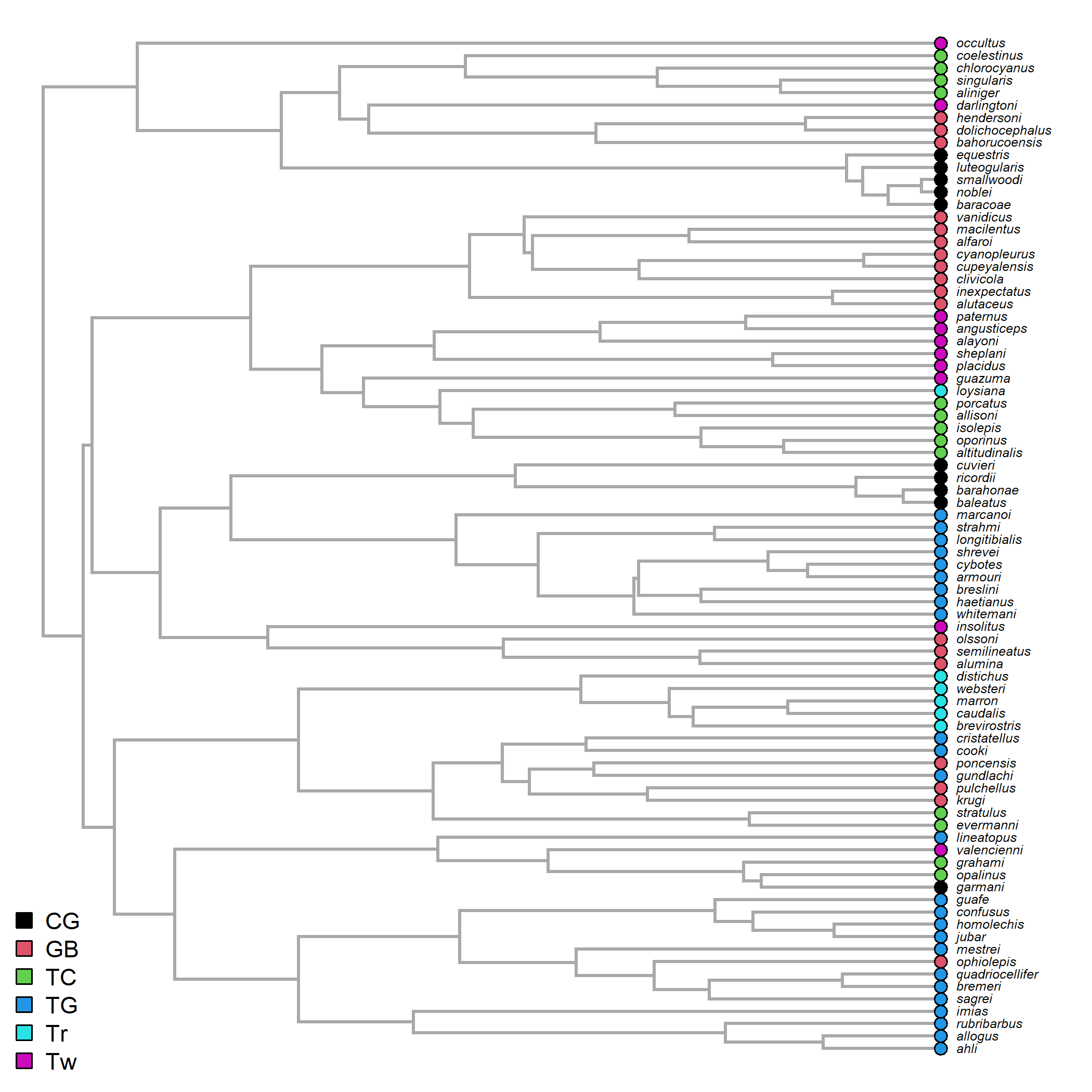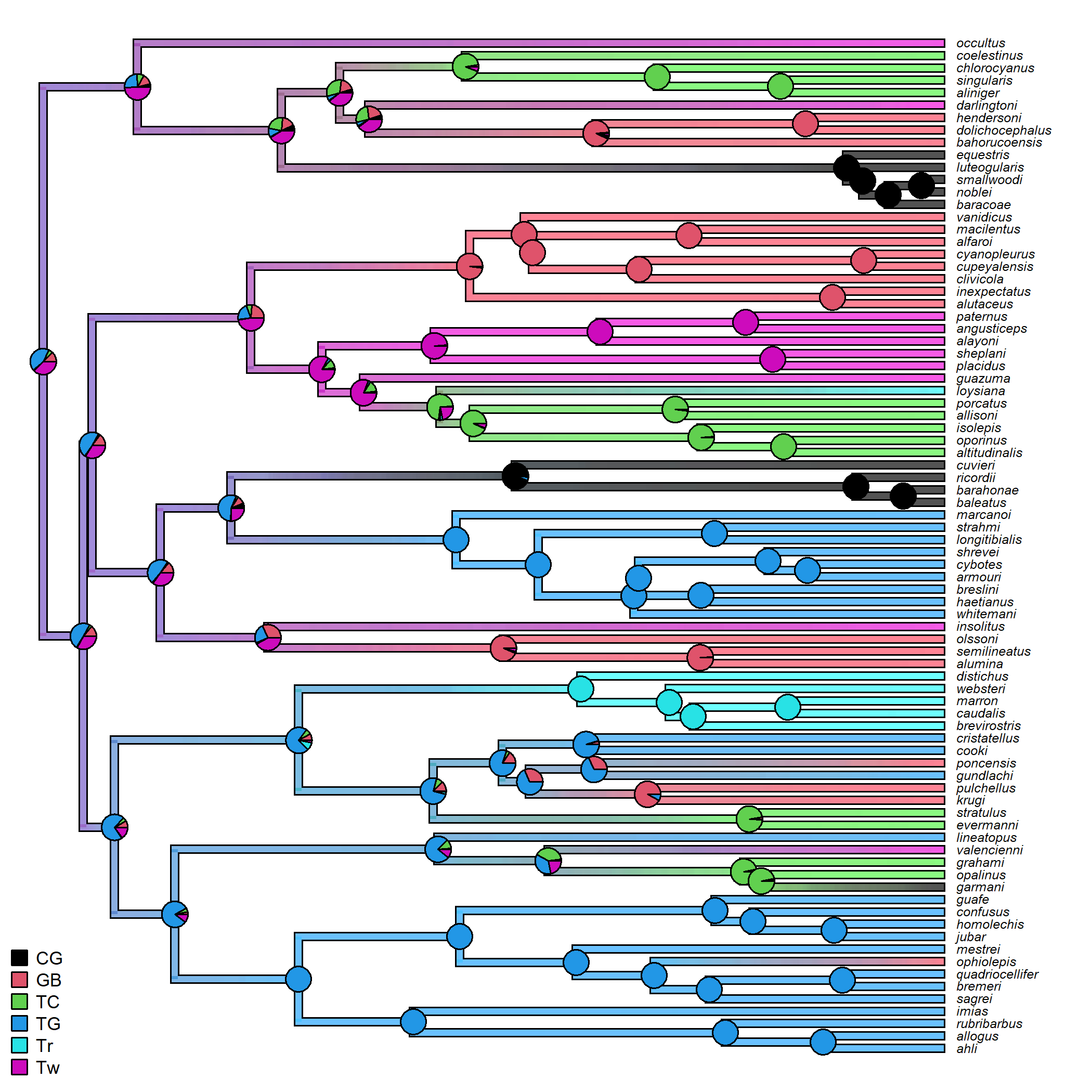## Friday, June 19, 2020

### Mapping a multi-state discrete character onto the edges of a tree using stochastic mapping & transparent colors

The phytools function `densityMap` is a handy tool for visualizing the posterior sample of character histories from stochastic mapping.

Unfortunately, it only works for a binary discrete trait - since what is being mapped is the probability of being in one of the two trait conditions. (The probability of being in the other trait condition is obviously just one minus this former value.)

It is, however, a relatively trivial matter to simultaneously visualize a set of stochastic mapped trees using transparent colors. Maybe if we combine this type of plot with posterior probabilities at nodes of the tree we might end up with a sensible visualization of uncertainty in discrete character ancestral state reconstruction on the phylogeny. What do you think?

Here's how to do it using Anolis ecomorph data.

To start, let's get & plot our data:

``````## first get our data:
library(phytools)
data(anoletree)
ecomorph<-as.factor(getStates(anoletree,"tips"))
anoletree<-as.phylo(anoletree)
cols<-setNames(palette()[1:length(levels(ecomorph))],
levels(ecomorph))
## then plot it:
plotTree(anoletree,ftype="i",fsize=0.5,color="darkgrey",
offset=0.5)
tiplabels(pie=to.matrix(ecomorph[anoletree\$tip.label],
levels(ecomorph)),piecol=cols,cex=0.3)
legend(x="bottomleft",levels(ecomorph),pch=22,
pt.bg=cols,pt.cex=1.5,bty="n",cex=0.9)
``````Next, let's do our stochastic mapping and then create a visualization of the posterior sample:

``````## stochastic mapping
map.trees<-make.simmap(anoletree,ecomorph,model="ER",
nsim=100)
``````
``````## make.simmap is sampling character histories conditioned on the transition matrix
##
## Q =
##             CG          GB          TC          TG          Tr          Tw
## CG -0.11570697  0.02314139  0.02314139  0.02314139  0.02314139  0.02314139
## GB  0.02314139 -0.11570697  0.02314139  0.02314139  0.02314139  0.02314139
## TC  0.02314139  0.02314139 -0.11570697  0.02314139  0.02314139  0.02314139
## TG  0.02314139  0.02314139  0.02314139 -0.11570697  0.02314139  0.02314139
## Tr  0.02314139  0.02314139  0.02314139  0.02314139 -0.11570697  0.02314139
## Tw  0.02314139  0.02314139  0.02314139  0.02314139  0.02314139 -0.11570697
## (estimated using likelihood);
## and (mean) root node prior probabilities
## pi =
##        CG        GB        TC        TG        Tr        Tw
## 0.1666667 0.1666667 0.1666667 0.1666667 0.1666667 0.1666667
``````
``````## Done.
``````
``````## next plot an outline tree:
plotTree(anoletree,ftype="i",fsize=0.5,offset=0.5,
lwd=6)
par(fg="transparent",lend=1)
plotTree(anoletree,ftype="i",fsize=0.5,offset=0.5,
## now plot our 100 stochastic map trees
## with 99% transparency
for(i in 1:length(map.trees)) plot(map.trees[[i]],
colors=sapply(cols,make.transparent,alpha=0.01),
par(fg="black")
nodelabels(pie=summary(map.trees)\$ace,piecol=cols,
cex=0.6)
legend(x="bottomleft",levels(ecomorph),pch=22,
pt.bg=cols,pt.cex=1.5,bty="n",cex=0.7)
``````That's OK….

One obvious problem with this kind of plot is that since we are not doing linear interpolation between two colors (or something equivalent in RGB color space), for edges that are really uncertain we can end up with a color combination that looks (or is) identical to the color of an entirely different state!

Nonetheless, when combinded with the marginal posterior probabilities at nodes it doesn't look like that is too common, even using this relatively simple default color scheme.

#### 1 comment:

1.This is awesome, Liam. Im trying it now!

Note: due to the very large amount of spam, all comments are now automatically submitted for moderation.